# Divide blend mode is a chromaticity-dependent editing operation

The Divide blend mode is a chromaticity-dependent editing operation and so gives different results in different RGB working spaces. Also, in the unbounded sRGB color space the Divide blend mode produces meaningless results when performed using image colors that are encoded using out of gamut channel values.

Written April 2014. Updated August 2014.

## Introduction: The Divide blend mode is a chromaticity dependent editing operation

### Division is the inverse of multiplication

Division is the inverse of multiplication, and multiplication is a chromaticity dependent editing operation, meaning the results of dividing one color by another color depend on the color space in which the division is done. So of course division is also a chromaticity dependent editing operation. Also, the Divide blend mode is designed to be used on in-gamut RGB values only. So in the unbounded sRGB color space the Divide blend mode produces meaningless results when performed using image colors that are encoded using out of gamut channel values.

### What the Divide blend mode is used for

This PhotoShop-oriented article has the best explanation I could find regarding specific uses of the Divide blend mode: The Fifth Group of Blend Modes. The Divide blend mode has very specific technical uses and also some artistic uses. One extremely important technical use of Divide is flat field removal. Artistic uses of Divide include producing line drawings and high key images.

Section B below gives an example showing that when used artistically rather than technically, results of using the Divide blend mode give different results in different working spaces. Which results are more pleasing of course is up to the artist to decide. Section C gives an example showing how the mathematics of Divide fails completely when a saturated image is converted from a wider gamut color space to unbounded sRGB. If the mathematics of Divide fail, any technical use of Divide also fails.

High bit depth GIMP 2.9 from git was used to prepare the images in the article. High bit depth GIMP 2.9 is a bit unusual among image editors because it does allow unbounded ICC profile conversions and image editing. The default GIMP 2.9 from git uses linear gamma RGB values for some editing operations and uses "gamma corrected" RGB values for other editing operations. For this article, to avoid accidental "apples to oranges" comparisions I used a version of GIMP 2.9 from git that I modified to ensure that all editing operations were done using linear gamma processing.

## Results of using the Divide blend mode to make a line drawing are chromaticity dependent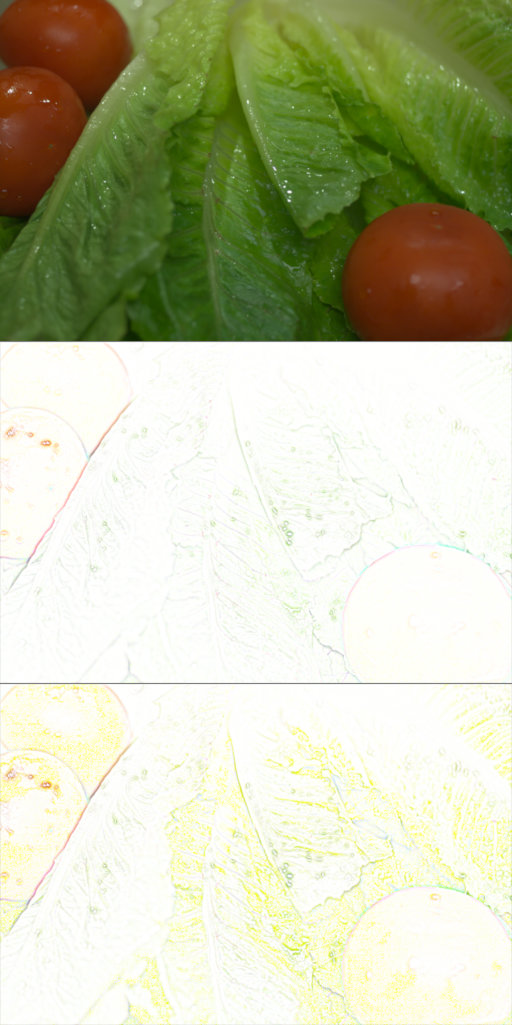Results from using the Divide blend mode to make a line drawing are chromaticity dependent: Top: a picture of some lettuce and tomatoes. The image was shot raw, developed with RawTherapee, and output in the ProPhotoRGB color space. Middle: Still in the ProPhotoRGB color space, I used Divide mode to create a line drawing. The procedure is simple: place a copy of the image over itself, blur the copy, and change the blend mode to Divide. Bottom: After converting the image to unbounded sRGB, the Divide blend mode gave the resulting line drawing a yellow color cast. As the artist I find the colors in the line drawing produced in the sRGB color space to be completely unacceptable. The Divide blend mode shouldn't have made the green lettuce and red tomatoes turn yellow.

## Dividing by red fails after the image is converted to unbounded sRGB

Dividing an image by an exact copy of itself produces solid white in any RGB working space. So if the only thing anyone ever did with the Divide blend mode is divide an image by itself to produce white, we could say that Divide blend mode works perfectly even after a saturated image is converted from a larger color gamut to unbounded sRGB.

However, dividing an image by itself to produce white is not an especially useful editing move, so let's divide an image layer by solid red (1.0, 0.0, 0.0) and see what happens. The test image in Figures 2 through 6 below is a composite of several colorful interpolated camera raw files, saved to disk in a custom camera-sized RGB working space which I'll refer to as "CameraRGB".

In normal bounded, display-referred image editing, dividing by the reddest possible red in any given color space sends the image's green and blue channels to 1.00 everywhere because the reddest possible red in any color space is (1.0, 0.0, 0.0). Dividing by zero in the green and blue channels sends those values to 1.0 because positive infinity, clipped to 1.0, is 1.0 (except in the case of solid red over solid black, the result is black, which seems odd). So in any display-referred RGB working space, the net result of dividing an image layer by that working space's reddest possible red is that the image becomes strongly cyan, only varying in intensity in the red channel. This is exactly what happens in the original CameraRGB color space: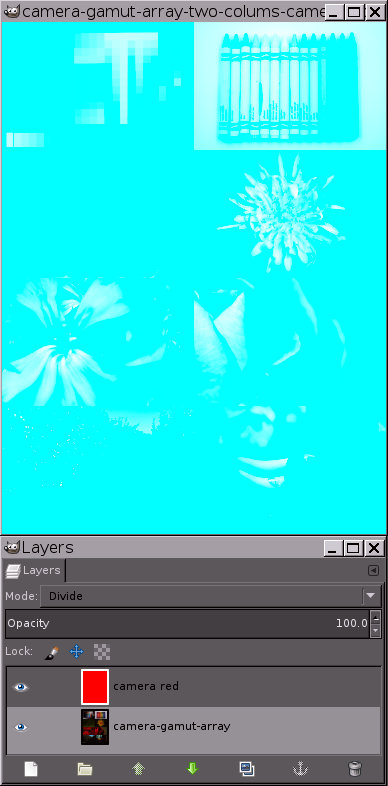In the CameraRGB working space, dividing the image by the reddest possible CameraRGB red (1.0, 0.0, 0.0) drives the Green and Blue channels to 1.0, producing a strong cyan cast.

When the image is converted to unbounded sRGB and divided by the same CameraRGB reddest red, which is encoded in the unbounded sRGB color space as (1.729899, -0.146738, -0.024542), as one would expect results are completely wrong. In fact the resulting RGB values represent entirely physically meaningless colors which the poor Color Management Module (LCMS2 in the present case) is doing it's best to display: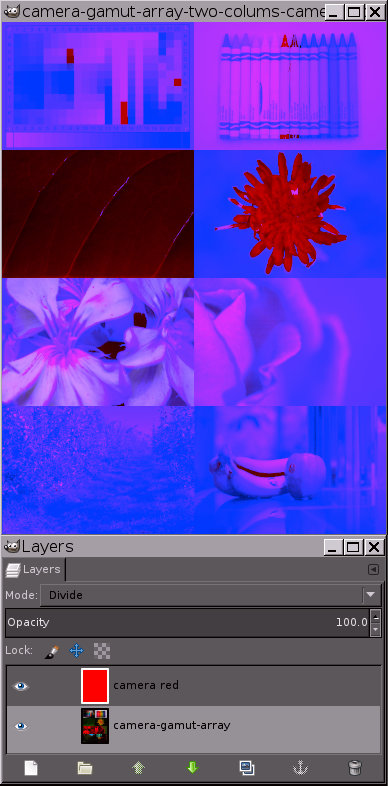After converting the image from the camera-sized color space to unbounded sRGB, dividing by the reddest possible camera red fails completely.

While still in the unbounded sRGB color space, dividing by the reddest possible bounded sRGB red instead of the reddest possible camera red also produces the wrong results: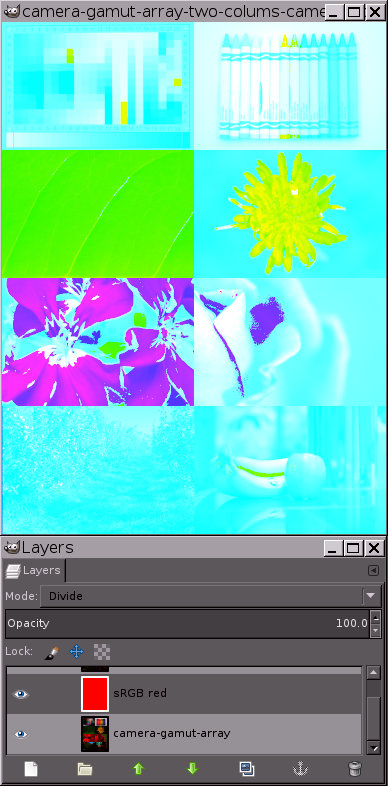In the un bounded sRGB color space, dividing the image by the reddest possible bounded sRGB red (1.0, 0.0, 0.0) also fails completely.

There is clipping code in the Divide blend mode algorithm that prevents the resulting RGB channel values from being less than zero or greater than one. One might suppose that removing the clipping code might help, it doesn't. Instead, removing the Divide blend mode clipping code completely destroys the functionality of the Divide blend mode even in the original camera space.

Here's the result of dividing the array of saturated images by CameraRGB red, in the CameraRGB working space, after the clipping code is removed: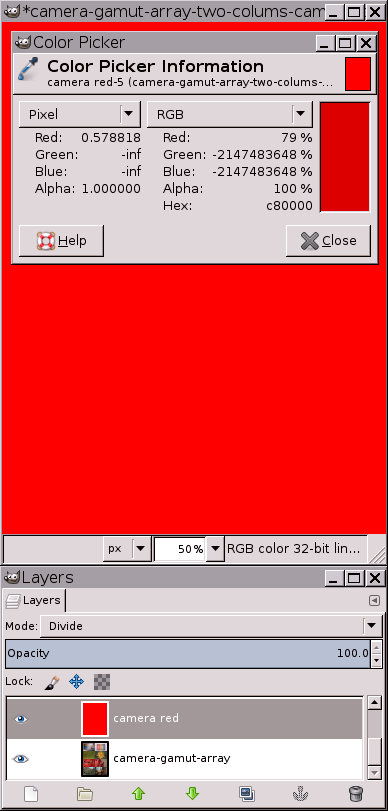After removing the Divide clipping code, dividing by CameraRGB red fails completely even in the original CameraRGB working space.

Here's the result of dividing the array of saturated images by CameraRGB red in the unbounded sRGB color space after the clipping code is removed: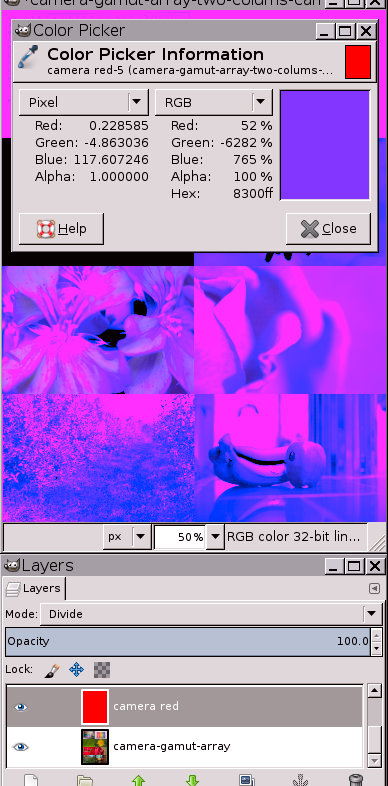After removing the Divide blend mode clipping code, dividing by red also fails completely after the image is converted to unbounded sRGB.

## Conclusion: Divide blend mode is a chromaticity dependent editing operation that can easily fail in unbounded RGB working spaces

The Divide blend mode is a chromaticity dependent editing operation, producing different colors in different RGB working spaces.

The Divide blend mode can be used artistically to make a line drawing. When making a line drawing of a photograph of green lettuce and red tomatoes, in the ProPhotoRGB color space, the space between the lines was almost white. In the unbounded sRGB color space, the same procedure produced a line drawing with a yellow color cast over much of the image.

The Divide blend mode assumes the image RGB values are in fact bounded by 0.0 and 1.0, which means technical uses of Divide fail completely after converting a saturated image from its original wider gamut color space to unbounded sRGB, producing meaningless results whenever either layer contains an out of gamut color.

### Test files

If you would like to check for yourself and you don't have any reasonably saturated interpolated camera raw files to play with, here are the image files and ICC profiles used in this demonstration: